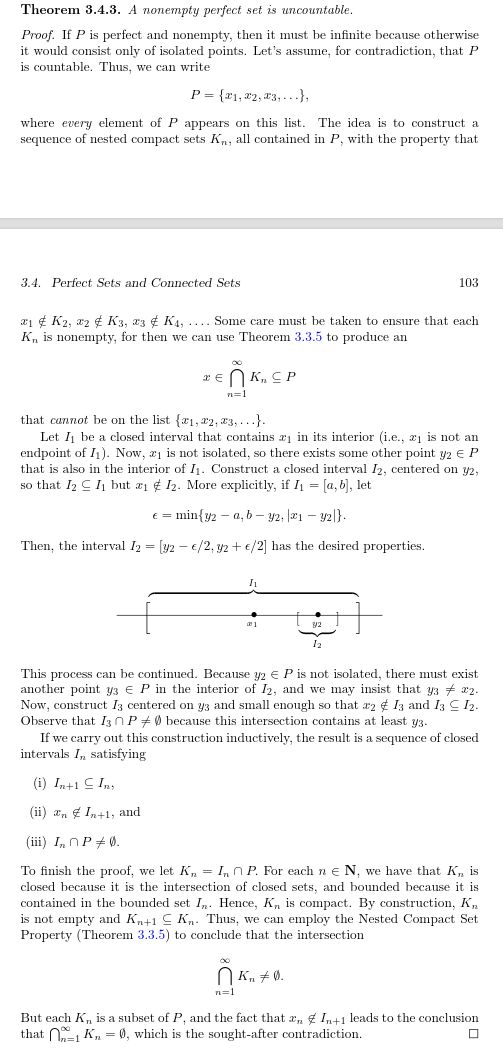Show Question
Math and science::Analysis

# Perfect Sets

The concept of a perfect set tries to generalize the idea of a closed interval whose end points are not equal.

### Perfect sets

A set $$P \subseteq \mathbb{R}$$ is perfect iff it is closed and contains no isolated points.

The following theorem highlights the importance of perfect sets. (Abbott actually introduces this theorem in order to motivate the concept of perfect sets. See the reverse side for more details.)

A nonempty perfect set is uncountable.

#### Motivation: a generalization

The nature of intervals that are closed and bounded allow for many interesting statements of the form:

if $$A \subseteq \mathbb{R}$$ is an interval that is closed and bounded, then the proposition $$P$$ holds.

For example, $$P$$ could be: all open covers have a finite sub-cover. What makes closed bounded intervals special can be generalized into the concept of compactness, and in doing so, we see that we can drop the requirements for such sets to be intervals at all–closed and bounded is sufficient.

In a similar way, consider the implication:

if $$A \subseteq \mathbb{R}$$ is a non-empty closed interval $$[a, b]$$, then it is uncountable.

It turns out that the antecedent can be generalized into the concept of perfect sets; A perfect set which is nonempty is uncountable.

### Proof

Copied directly from Abbott:### Example

Some examples:

• Closed intervals are perfect (other than singleton sets, $$[a, a]$$).
• The Cantor set is perfect.
• What else?

#### Source

Abbott, p102 (2nd edition)